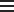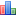Short Citation:
Pacific Islands Fisheries Science Center, 2023: Detailed Information on longline data collected during NOAA research cruises in 1950-1960, 1962-1964, 1966-1970, and 1973, https://www.fisheries.noaa.gov/inport/item/5872.

## Item Identification

 Title: Detailed Information on longline data collected during NOAA research cruises in 1950-1960, 1962-1964, 1966-1970, and 1973 RP024 Longlining #3 Detailed Information on Longline Deck #3 (Revised) (RSCH) Completed 2002-10-17 2022-06-15 This data set contains catch (numbers) and effort data from RP022 and RP023 data collected during NOAA research cruises. Date, latitude, longitude, float line length, mainline length, gear type, number of hooks per basket, number of baskets fished, number of hooks fished and number of various tunas, sharks, marlins and fishes caught are included in this dataset. Research Record count: 1,672 SOURCE ID: RP024

## Keywords

### Theme Keywords

Thesaurus Keyword
UNCONTROLLED
None LONGLINE
None PIFSC
None PIFSC Historical
None RP
None SIS

## Physical Location

 Organization: Pacific Islands Fisheries Science Center Honolulu HI USA

## Data Set Information

 Data Set Scope Code: Data Set Oracle Database None Planned Table (digital) While every effort has been made to ensure that these data are accurate and reliable within the limits of the current state of the art, NOAA cannot assume liability for any damages caused by errors or omissions in the data, nor as a result of the failure of the data to function on a particular system. NOAA makes no warranty, expressed or implied, nor does the fact of distribution constitute such a warranty.

## Support Roles

### Data Steward

CC ID: 775401
 Date Effective From: 2018 Miyamoto, Brent M 1845 Wasp Blvd. Honolulu, HI 96818 USA brent.miyamoto@noaa.gov 808-725-5340 8:15 a.m. - 5:15 p.m. HST

### Distributor

CC ID: 775402
 Date Effective From: 2018 Pacific Islands Fisheries Science Center (PIFSC) 1845 Wasp Blvd. Honolulu, HI 96818 USA pifsc.info@noaa.gov 808-725-5360 https://www.pifsc.noaa.gov 8:00 a.m. - 4:30 p.m.

CC ID: 775403
 Date Effective From: 2018 Miyamoto, Brent M 1845 Wasp Blvd. Honolulu, HI 96818 USA brent.miyamoto@noaa.gov 808-725-5340 8:15 a.m. - 5:15 p.m. HST

### Point of Contact

CC ID: 775404
 Date Effective From: 2018 Tokita, Christopher Y 1845 Wasp Blvd. Honolulu, HI 96818 USA christopher.tokita@noaa.gov (808)725-5342 8:00 a.m. - 4:30 p.m. HST M-F

## Extents

 Currentness Reference: Ground Condition

### Extent Group 1 / Geographic Area 1

CC ID: 1176788
 W° Bound: 159.867 -110.15 47.5167 -24.3667

### Extent Group 1 / Time Frame 1

CC ID: 236659
 Time Frame Type: Range 1950 1973 1950-1960, 1962-1964, 1966-1970, 1973

## Access Information

 Security Class: Unclassified Send written request to PIFSC. None None None

## Distribution Information

### Distribution 1

CC ID: 1176364
 Start Date: 2022-06-22 Present https://oceanwatch.pifsc.noaa.gov/xfer/PIFSC_PIRO_bulk_data_download_InPort_5872.tgz Pacific Islands Fisheries Science Center (PIFSC) (2018 - Present) csv (comma-separated values)

## Issues

### Issue 1

CC ID: 1171476
 Issue Date: 2022-05-16 Miyamoto, Brent M RESOLVED ISSUES: DUPLICATE SEQUENCE NUMBERS: 1) VESSEL_CODE=007, CRUISE_NUMBER=0057, DATE=14-JUN-1962, STATION_NUMBER='007', SEQUENCE_NUMBER = 00000007 => 00000009 2) VESSEL_CODE=007, CRUISE_NUMBER=0057, DATE=14-JUN-1962, STATION_NUMBER='007', SEQUENCE_NUMBER = 00000008 => 00000010 SPECIES COUNTS (DATA SHIFTED) 1) VESSEL_CODE=005, CRUISE_NUMBER=0025, DATE=15-MAY-1955, STATION_NUMBER='007', SEQUENCE_NUMBER=00000026, NUMBER_OF_SILKY_SHARK = ' 0' => NULL 2) VESSEL_CODE=005, CRUISE_NUMBER=0025, DATE=15-MAY-1955, STATION_NUMBER='007', SEQUENCE_NUMBER=00000026, NUMBER_OF_GREAT_BLUE_SHARK = '6' => '06' OCTANT 1) VESSEL_CODE=005, CRUISE_NUMBER=0011, DATE=19-FEB-1952, STATION_NUMBER='003', SEQUENCE_NUMBER=00000016, OCTANT = 6 => 7 (FOR LONGITUDE DEGREES 180) 2) VESSEL_CODE=005, CRUISE_NUMBER=0011, DATE=19-FEB-1952, STATION_NUMBER='003', SEQUENCE_NUMBER=00000017, OCTANT = 6 => 7 (FOR LONGITUDE DEGREES 180)

### Issue 2

CC ID: 1171643
 Issue Date: 2022-05-18 Miyamoto, Brent M UNRESOLVED ISSUE: OCTANT - 5/18/2022 VESSEL_CODE=005, CRUISE_NUMBER=0011 1) DATE=10-MAR-1952, STATION_NUMBER=25, SEQUENCE_NUMBER IN (00000039, 00000040), OCTANT = 6. BECAUSE THE SOURCE OF LATITUDE AND LONGITUDE ARE NOT KNOWN, WILL LEAVE THE OCTANT AS 6 (SW). HOWEVER, THE RP022 DATA COLLECTION FORM AND NARRATIVE REPORT INDICATE OCTANT 1 (NW). 2) DATE=11-MAR-1952, STATION_NUMBER=25, SEQUENCE_NUMBER IN (00000041, 00000042), OCTANT = 6. BECAUSE THE SOURCE OF LATITUDE AND LONGITUDE ARE NOT KNOWN, WILL LEAVE THE OCTANT AS 6 (SW). HOWEVER, THE RP022 DATA COLLECTION FORM AND NARRATIVE REPORT INDICATE OCTANT 1 (NW). 3) DATE=12-MAR-1952, STATION_NUMBER=27, SEQUENCE_NUMBER=00000043, OCTANT = 6. BECAUSE THE SOURCE OF LATITUDE AND LONGITUDE ARE NOT KNOWN, WILL LEAVE THE OCTANT AS 6 (SW). HOWEVER, THE RP022 DATA COLLECTION FORM AND NARRATIVE REPORT INDICATE OCTANT 1 (NW).

### Issue 3

CC ID: 1176134
 Issue Date: 2022-06-13 Miyamoto, Brent M RESOLVED ISSUE: NUMBER_BLACK_TUNA / NUMBER_MAKO (BONITO) SHARK - 2022-06-13 This column actually contains the counts for the NUMBER_MAKO (BONITO)_SHARK or NUMBER_MACKEREL_SHARK (SEE OTHER ISSUE. NUMBER_MAKO_SHARK: 1) VESSEL_CODE=004, CRUISE_NUMBER=0007, STATION_NUMBER=027, DATE=18-NOV-1950, SEQUENCE_NUMBER=00000019, NUMBER_MAKO_SHARK=01 2) VESSEL_CODE=004, CRUISE_NUMBER=0018, STATION_NUMBER=032, DATE=11-NOV-1952, SEQUENCE_NUMBER=00000022, NUMBER_MAKO_SHARK=01 3) VESSEL_CODE=004, CRUISE_NUMBER=0029, STATION_NUMBER=001, DATE=05-MAY-1955, SEQUENCE_NUMBER=00000001, NUMBER_MAKO_SHARK=01 4) VESSEL_CODE=004, CRUISE_NUMBER=0029, STATION_NUMBER=005, DATE=07-MAY-1955, SEQUENCE_NUMBER=00000005, NUMBER_BONITO_SHARK=01 5) VESSEL_CODE=004, CRUISE_NUMBER=0029, STATION_NUMBER=007, DATE=08-MAY-1955, SEQUENCE_NUMBER=00000007, NUMBER_MAKO_SHARK=01 6) VESSEL_CODE=004, CRUISE_NUMBER=0029, STATION_NUMBER=042, DATE=03-JUN-1955, SEQUENCE_NUMBER=00000039, NUMBER_MAKO_SHARK=01 7) VESSEL_CODE=004, CRUISE_NUMBER=0046, STATION_NUMBER=070, DATE=20-AUG-1958, SEQUENCE_NUMBER=00000004, NUMBER_MAKO_SHARK=01 8) VESSEL_CODE=004, CRUISE_NUMBER=0051, STATION_NUMBER=006, DATE=13-MAR-1959, SEQUENCE_NUMBER=00000001, NUMBER_MAKO-SHARK=01 9) VESSEL_CODE=005, CRUISE_NUMBER=0011, STATION_NUMBER=0001, DATE=29-JAN-1952, SEQUENCE_NUMBER=00000001, NUMBER_MAKO_SHARK=01 10) VESSEL_CODE=005, CRUISE_NUMBER=0011, STATION_NUMBER=002, DATE=30-JAN-1952, SEQUENCE_NUMBER=00000002, NUMBER_BONITO_SHARK=01 11) VESSEL_CODE=005, CRUISE_NUMBER=0014, STATION_NUMBER=007, DATE=03-FEB-1953, SEQUENCE_NUMBER=00000011, NUMBER_MAKO_SHARK=01 12) VESSEL_CODE=005, CRUISE_NUMBER=0015, STATION_NUMBER=005, DATE=05-MAY-1953, SEQUENCE_NUMBER=00000007, NUMBER_MAK_SHARK=01 13) VESSEL_CODE=005, CRUISE_NUMBER=0015, STATION_NUMBER=006, DATE=06-MAY-1953, SEQUENCE_NUMBER=00000008, NUMBER_MAKO_STATION=01 14) VESSEL_CODE=005, CRUISE_NUMBER=0015, STATION_NUMBER=008, DATE=08-MAY-1953, SEQUENCE_NUMBER=00000009, NUMBER_MAKO_SHARK=01 15) VESSEL_CODE=005, CRUISE_NUMBER=0015, STATION_NUMBER=011, DATE=11-MAY-1953, SEQUENCE_NUMBER=00000018, NUMBER_MAKO_SHARK=01 16) VESSEL_CODE=005, CRUISE_NUMBER=0015, STATION_NUMBER=014, DATE=14-MAY-1953, SEQUENCE_NUMBER=00000024, NUMBER_ MAKO_SHARK=01 17) VESSEL_CODE=005, CRUISE_NUMBER=0015, STATION_NUMBER=022, DATE=02-JUN-1953, SEQUENCE_NUMBER=00000040, NUMBER_MAKO_SHARK=01 18) VESSEL_CODE=005, CRUISE_NUMBER=0016, STATION_NUMBER=027, DATE=24-AUG-1953, SEQUENCE_NUMBER=00000014, NUMBER_MAKO_SHARK=01 19) VESSEL_CODE=005, CRUISE_NUMBER=0016, STATION_NUMBER=029, DATE=26-AUG-1953, SEQUENCE_NUMBER=00000020, NUMBER_MAKO_SHARK=01 20) VESSEL_CODE=005, CRUISE_NUMBER=0016, STATION_NUMBER=030, DATE=27-AUG-1953, SEQUENCE_NUMBER=00000023, NUMBER_MAKO_SHARK=01 21) VESSEL_CODE=005, CRUISE_NUMBER=0018, STATION_NUMBER=010, DATE=04-DEC-1953, SEQUENCE_NUMBER=00000008, NUMBER_MAKO_SHARK=01 22) VESSEL_CODE=005, CRUISE_NUMBER=0019, STATION_NUMBER=003, DATE=22-JAN-1954, SEQUENCE_NUMBER=00000005, NUMBER_BONITO_SHARK=02 23) VESSEL_CODE=005, CRUISE_NUMBER=0019, STATION_NUMBER=005, DATE=24-JAN-1954, SEQUENCE_NUMBER=00000009, NUMBER_BONITO_SHARK=01 24) VESSEL_CODE=005, CRUISE_NUMBER=0019, STATION_NUMBER=008, DATE=27-JAN-1954, SEQUENCE_NUMBER=00000015, NUMBER_BONITO_SHARK=01 25) VESSEL_CODE=005, CRUISE_NUMBER=0022, STATION_NUMBER=011, DATE=21-SEP-1954, SEQUENCE_NUMBER=00000011, NUMBER_BONITO_SHARK=02 26) VESSEL_CODE=005, CRUISE_NUMBER=0022, STATION_NUMBER=013, DATE=22-SEP-1954, SEQUENCE_NUMBER=00000013, NUMBER_MAKO_SHARK=01 27) VESSEL_CODE=005, CRUISE_NUMBER=0022, STATION_NUMBER=017, DATE=02-OCT-1954, SEQUENCE_NUMBER=00000020, NUMBER_MAKO_SHARK=01 28) VESSEL_CODE=007, CRUISE_NUMBER=0015, STATION_NUMBER=009, DATE=23-FEB-1954, SEQUENCE_NUMBER=00000011, NUMBER_MAKO_SHARK=01 29) VESSEL_CODE=007, CRUISE_NUMBER=0015, STATION_NUMBER=017, DATE=04-MAR-1954, SEQUENCE_NUMBER=00000018, NUMBER_MAKO_SHARK=01

### Issue 4

CC ID: 1176135
 Issue Date: 2022-06-13 Miyamoto, Brent M RESOLVED ISSUE: NUMBER_BLACK_TUNA / NUMBER_MACKEREL_SHARK - 2022-06-13 This column actually contains the counts for the NUMBER_MAKO (BONITO)_SHARK or NUMBER_MACKEREL_SHARK. NUMBER_MACKEREL_SHARK: 1) VESSEL_CODE=004, CRUISE_NUMBER=0007, STATION_NUMBER=033, DATE=25-NOV-1950, SEQUENCE_NUMBER=00000025, NUMBER_MACKEREL_SHARK=002 2) VESSEL_CODE=004, CRUISE_NUMBER=0007, STATION_NUMBER=034, DATE=26-NOV-1950, SEQUENCE_NUMBER=00000026, NUMBER_MACKEREL_SHARK=004

### Issue 5

CC ID: 1176200
 Issue Date: 2022-06-15 Miyamoto, Brent M RESOLVED ISSUE: TOTAL_NUMBER_HOOKS_FISHED 1) VESSEL_CODE=007, CRUISE_NUMBER=0023, STATION_NUMBER=016, DATE=30-SEP-1955, SEQUENCE_NUMBER=00000002, TOTAL_NUMBER_HOOKS_FISHED = 260 => 247; FOR THIS TRIP, COUNTS OF THE NUMBER OF HOOKS RETRIEVED WERE ENTERED INTO TOTAL_NUMBER_HOOKS_FISHED

## Technical Environment

Description: Oracle

## Data Quality

Quality Control Procedures Employed: QC review prior to data entry. Further QC after data entry.

## Data Management

 Have Resources for Management of these Data Been Identified?: Yes Unknown No No 1 year NCEI-MD 1 year PIFSC ITS performs scheduled back ups.

## Lineage

Lineage Statement: Data were collected in the field on PIFSC data collection forms, and data entry was conducted at PIFSC to create ASCII fixed record length flat files. The flat files were then loaded into an Oracle database.

## Child Items

Rubric scores updated every 15mType Title
Entity RP024AA1_RSCH_V

## Catalog Details

 Catalog Item ID: 5872 gov.noaa.nmfs.inport:5872 Christopher Y Tokita 2007-10-24 12:35+0000 SysAdmin InPortAdmin 2022-10-20 02:17+0000 2022-06-17 PIFSC Published Externally N 2022-06-17 1 Year 2023-06-17